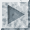[an error occurred while processing this directive]

## quantifiersquantifiers: In techspeak and jargon, the standard metric prefixes used in the SI (Syst`eme International) conventions for scientific measurement have dual uses. With units of time or things that come in powers of 10, such as money, they retain their usual meanings of multiplication by powers of 1000 = 10^3. But when used with bytes or other things that naturally come in powers of 2, they usually denote multiplication by powers of 1024 = 2^(10).

Here are the SI magnifying prefixes, along with the corresponding binary interpretations in common use:

```     prefix  decimal  binary
kilo-   1000^1   1024^1 = 2^10 = 1,024
mega-   1000^2   1024^2 = 2^20 = 1,048,576
giga-   1000^3   1024^3 = 2^30 = 1,073,741,824
tera-   1000^4   1024^4 = 2^40 = 1,099,511,627,776
peta-   1000^5   1024^5 = 2^50 = 1,125,899,906,842,624
exa-    1000^6   1024^6 = 2^60 = 1,152,921,504,606,846,976
zetta-  1000^7   1024^7 = 2^70 = 1,180,591,620,717,411,303,424
yotta-  1000^8   1024^8 = 2^80 = 1,208,925,819,614,629,174,706,176
```
Here are the SI fractional prefixes:

```     *prefix  decimal     jargon usage*
milli-  1000^-1     (seldom used in jargon)
micro-  1000^-2     small or human-scale (see micro-)
nano-   1000^-3     even smaller (see nano-)
pico-   1000^-4     even smaller yet (see pico-)
femto-  1000^-5     (not used in jargon--yet)
atto-   1000^-6     (not used in jargon--yet)
zepto-  1000^-7     (not used in jargon--yet)
yocto-  1000^-8     (not used in jargon--yet)
```
The prefixes zetta-, yotta-, zepto-, and yocto- have been included in these tables purely for completeness and giggle value; they were adopted in 1990 by the `19th Conference Generale des Poids et Mesures'. The binary peta- and exa- loadings, though well established, are not in jargon use either -- yet. The prefix milli-, denoting multiplication by 1/1000, has always been rare in jargon (there is, however, a standard joke about the `millihelen' -- notionally, the amount of beauty required to launch one ship). See the entries on micro-, pico-, and nano- for more information on connotative jargon use of these terms. `Femto' and `atto' (which, interestingly, derive not from Greek but from Danish) have not yet acquired jargon loadings, though it is easy to predict what those will be once computing technology enters the required realms of magnitude (however, see attoparsec).

There are, of course, some standard unit prefixes for powers of 10. In the following table, the `prefix' column is the international standard suffix for the appropriate power of ten; the `binary' column lists jargon abbreviations and words for the corresponding power of 2. The B-suffixed forms are commonly used for byte quantities; the words `meg' and `gig' are nouns that may (but do not always) pluralize with `s'.

```     prefix   decimal   binary       pronunciation
kilo-       k      K, KB,       /kay/
mega-       M      M, MB, meg   /meg/
giga-       G      G, GB, gig   /gig/,/jig/
```
Confusingly, hackers often use K or M as though they were suffix or numeric multipliers rather than a prefix; thus "2K d The correct size is 1440 KB, that is, 1440 * 1024 = 1474560 bytes. So the `mega' in `1.44 MB' is compounded of two `kilos', one of which is 1024 and the other of which is 1000. The correct number of megabytes would of course be 1440 / 1024 = 1.40625. Alas, this fine point is probably lost on the world forever.

[1993 update: hacker Morgan Burke has proposed, to general approval on Usenet, the following additional prefixes:

groucho 10^(-30) harpo 10^(-27) harpi 10^(27) grouchi 10^(30)

We observe that this would leave the prefixes zeppo-, gummo-, and chico- available for future expansion. Sadly, there is little immediate prospect that Mr. Burke's eminently sensible proposal will be ratified.][Image_Link]../gi The correct size is 1440 KB, that is, 1440 * 1024 = 1474560 bytes. So the `mega' in `1.44 MB' is compounded of two `kilos', one of which is 1024 and the other of which is 1000. The correct number of megabytes would of course be 1440 / 1024 = 1.40625. Alas, this fine point is probably lost on the world forever.

[1993 update: hacker Morgan Burke has proposed, to general approval on Usenet, the following additional prefixes:

groucho 10^(-30) harpo 10^(-27) harpi 10^(27) grouchi 10^(30)

We observe that this would leave the prefixes zeppo-, gummo-, and chico- available for future expansion. Sadly, there is little immediate prospect that Mr. Burke's eminently sensible proposal will be ratified.]